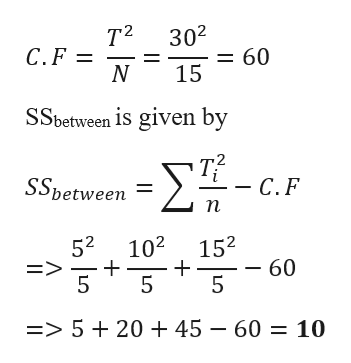# A research study comparing three treatments with n = 5 in each treatment produces T1 = 5, T2 = 10, T3 = 15, with SS1 = 6, SS2 = 9, and SX2 (to the power 2) = 94. For this study, what is SSbetween?

Question
18 views

A research study comparing three treatments with n = 5 in each treatment produces T1 = 5, T2 = 10, T3 = 15, with SS1 = 6, SS2 = 9, and SX2 (to the power 2) = 94. For this study, what is SSbetween?

check_circle

Step 1

Given Data

n = 5

T1 = 5

T2 = 10

T3 = 15

SS1 = 6

SS2 = 9

SS3 = 9

SX2  = 94

n = 5

Treatments (N) = 3 x 5 = 15

Total Treatments T = T1 + T2 + T3 = 5 + 10 + 15 = 30

C...help_outlineImage TranscriptioncloseT2 302 C. F N = 60 15 SSbetween is given by ΣΕ-, SSbetween - C.F n 52 E> 5 102 152 - 60 5 => 52045 - 60 10 fullscreen

### Want to see the full answer?

See Solution

#### Want to see this answer and more?

Solutions are written by subject experts who are available 24/7. Questions are typically answered within 1 hour.*

See Solution
*Response times may vary by subject and question.
Tagged in

### Other